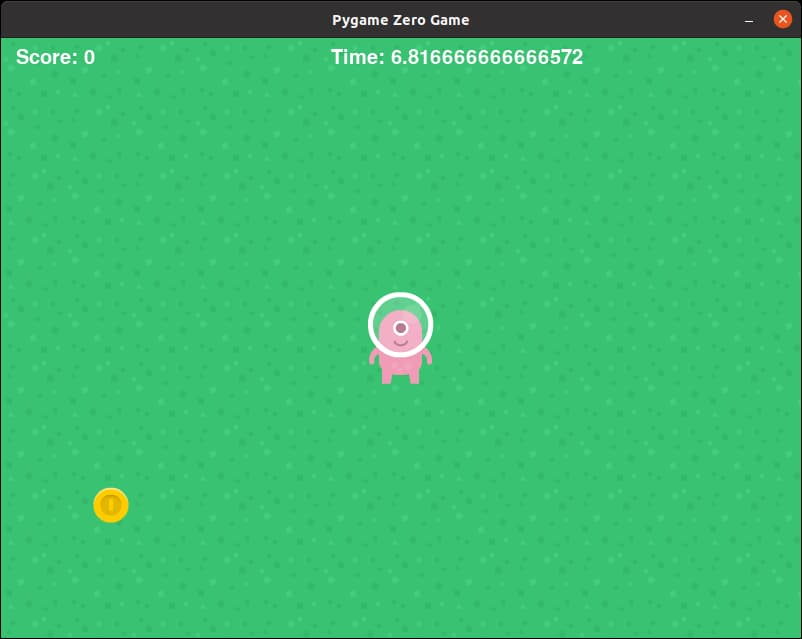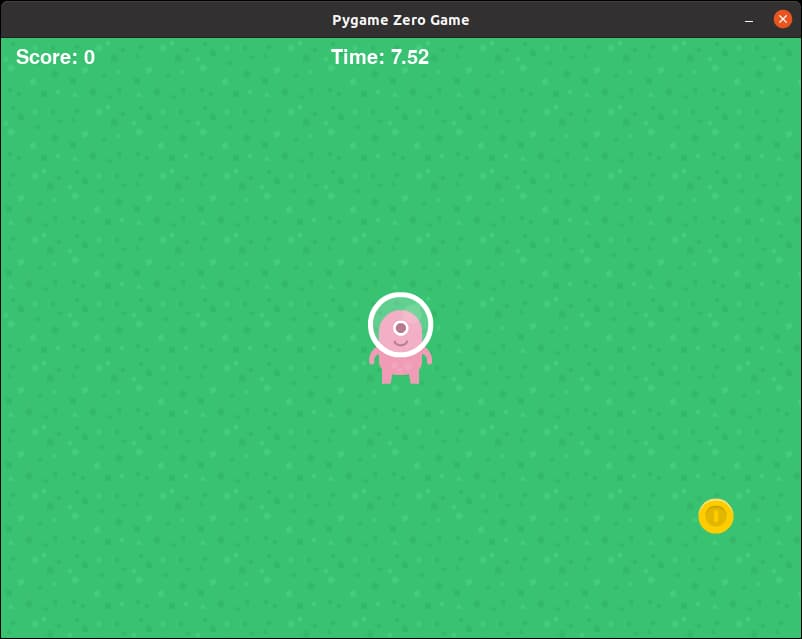# Timer

We want to add a timer that counts down to zero. When the time is up, we'll end the game and display the score.

Create another global variable and start it to 10, representing 10 seconds, at the start of the game.

``````timer = 10
``````

Since the update() function runs 60 times per second, we will subtract 1/60th of a second in every update(). Since timer is a global variable, we'll need declare that it is global first.

``````global timer

timer -= 1 / 60
``````

## Display the Timer

Just like the score, we will use the `screen.draw.text()` function, but we'll draw the time in a different position. Again, this needs to be in draw().

``````screen.draw.text('Time: ' + str(timer), (330,10), color=(255,255,255), fontsize=30)
``````Ok. We got the timer now, but that's a lot of decimal places. Let's round the timer value to 2 decimal places before we convert it to a string. Change str(timer) into...

``````str(round(timer, 2))
``````Much better now!

## At this point...

Right now your code should look like this (new lines are highlighted in yellow)...

``````import pgzrun
import random

WIDTH = 800
HEIGHT = 600

background = Actor('grass')

player = Actor('p3_front')
player.x = 400
player.y = 300

coin = Actor('coingold')
coin.x = random.randint(0, 800)
coin.y = random.randint(0, 600)

score = 0
timer = 10

def update():
global score
global timer

timer -= 1 / 60

if keyboard.up:
player.y -= 5
if keyboard.down:
player.y += 5
if keyboard.left:
player.x -= 5
if keyboard.right:
player.x += 5

if player.colliderect(coin):
coin.x = random.randint(0, 800)
coin.y = random.randint(0, 600)
score += 1

def draw():
background.draw()
player.draw()
coin.draw()
screen.draw.text('Score: ' + str(score), (15,10), color=(255,255,255), fontsize=30)
screen.draw.text('Time: ' + str(round(timer, 2)), (330,10), color=(255,255,255), fontsize=30)

pgzrun.go() # Must be last line
``````